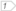#Boost C++ Libraries

...one of the most highly regarded and expertly designed C++ library projects in the world.

##### expand (with strategy)

Expands (with strategy)

###### Synopsis

```template<typename Box, typename Geometry, typename Strategy>
void expand(Box & box, Geometry const & geometry, Strategy const & strategy)```

###### Parameters

Type

Concept

Name

Description

Box &

type of the box

box

box to be expanded using another geometry, mutable

Geometry const &

Any type fulfilling a Geometry Concept

geometry

A model of the specified concept geometry which envelope (bounding box)

Strategy const &

Any type fulfilling a expand Strategy Concept

strategy

The strategy which will be used for expand calculations will be added to the box

Either

`#include <boost/geometry.hpp>`

Or

`#include <boost/geometry/algorithms/expand.hpp>`

###### Conformance

The function expand is not defined by OGC.

###### Behavior

Case

Behavior

Box / Point

Box is expanded to include the specified Point

Box / Box

Box is expanded to include the specified Box

Box / Other geometries

Not yet supported in this version

NoteTo use expand with another geometry type then specified, use expand(make_envelope(geometry)

Linear

###### Example

Shows the usage of expand

```#include <iostream>
#include <list>

#include <boost/geometry.hpp>
#include <boost/geometry/geometries/box.hpp>
#include <boost/geometry/geometries/point_xy.hpp>

int main()
{
typedef boost::geometry::model::d2::point_xy<short int> point_type;
typedef boost::geometry::model::box<point_type> box_type;

using boost::geometry::expand;

box_type box = boost::geometry::make_inverse<box_type>();expand(box, point_type(0, 0));
expand(box, point_type(1, 2));
expand(box, point_type(5, 4));
expand(box, boost::geometry::make<box_type>(3, 3, 5, 5));

std::cout << boost::geometry::dsv(box) << std::endl;

return 0;
}
```

 expand is usually preceded by a call to assign_inverse or make_inverse

Output:

```((0, 0), (5, 5))
```